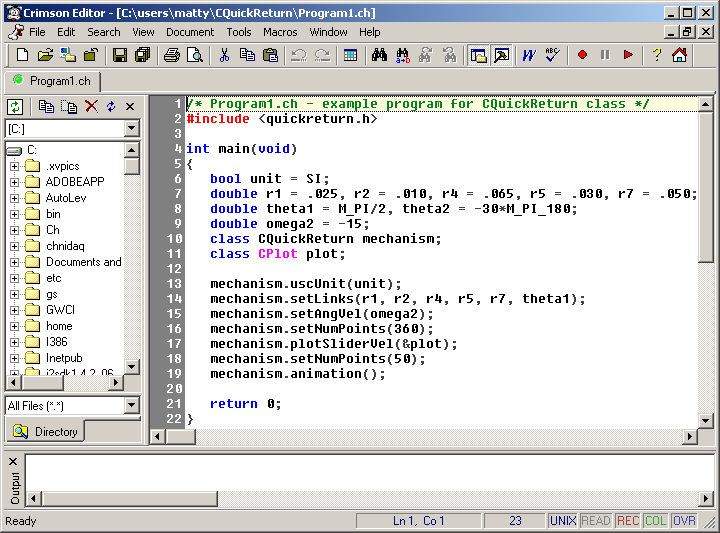## Example Design of a Withworth Quick Return Mechanism

### Using Ch mechanism toolkit and the code available from this website

#### Problem Statement

With the specifications for the mechanism given here, plot the velocity of the output slider versus time and display an animation of the mechanism in action. the links have the following lengths:
r1 = 25 mm,   r2 = 10 mm,   r4 = 65 mm,   r5 = 30 mm,   r7 = 50 mm
The ground link, r1, will be at 90°. The angular velocity of the input link is -15 rads per sec.#### Design specifications

To solve the problem we'll use the `CQuickReturn` class that was developed to analyze this type of mechanism. It has member functions to enter the mechanism specifications and others that present the output to the user. The code shown below uses this class.

#include <quickreturn.h> int main(void) { bool unit = SI; double r1 = .025, r2 = .010, r4 = .065, r5 = .030, r7 = .050; double theta1 = M_PI/2, theta2 = -30*M_PI_180; double omega2 = -15; class CQuickReturn mechanism; class CPlot plot; mechanism.uscUnit(unit); mechanism.setLinks(r1, r2, r4, r5, r7, theta1); mechanism.setAngVel(omega2); mechanism.setNumPoints(360); mechanism.plotSliderVel(&plot); mechanism.setNumPoints(50); mechanism.animation(); return 0; }This code can be run using the Crimson Editor as an Integrated Development Environment (IDE) shown above. It allows the execution of Ch programs from this GUI and is free to download. Below we describe how the code interacts with the member functions in the class. The first line of the program

#include <quickreturn.h>

includes the header file `quickreturn.h` which defines the CQuickReturn class, macros, and prototypes of member functions. Like all C/C++ programs, the program is started with the `main()` function. The next four lines

unit = SI; double r1 = .025, r2 = .010, r4 = .065, r5 = .030, r7 = .050; double theta1 = M_PI/2, theta2 = -30*M_PI_180; double omega2 = -15;

define the unit type, link lengths, ground and input link angles (in rad), and input link angular velocity (in rad/s) of the quick return mechanism. The lines

class CQuickReturn mechanism; class CPlot plot;

construct an object of the `CQuickReturn` class for the calculations and the `CPlot` class to display the output. The following three lines

mechanism.uscUnit(unit); mechanism.setLinks(r1, r2, r4, r5, r7, theta1); mechanism.setAngVel(omega2);

set the units, dimensions of each link, phase angle for link 1, and velocity of the input link as defined above. One line

mechanism.setNumPoints(360);

is needed to set the number of points to be used for plotting. The line

mechanism.plotSliderVel(&plot);

plots the velocity profile of the output slider, link 6. Figure 9 shows the velocity profile of the output slider after Program 1 has been executed. The last two lines

mechanism.setNumPoints(50); mechanism.animation();

reset the number of points and create an animation shown below. Fewer points are used because the animation doesn't need as many as a plot to create decent output.

The results of running the above program are displayed below. The first is the velocity profile for the slider versus the time. The animation output is also shown.#### Try the code yourself on the Web or your own computer

To try out this analysis on the Web, you can use the Web-based interface to this code. The animation requires installation of Ch and its Mechanism Toolkit on your computer. The code that creates the class for the analysis of the Withworth quick return mechanisms can be obtained on the main page. It will allow you to run the analyses shown here on your own computer. It requires the same software, Ch and the Mechanism ToolKit.

The Ch software is free for academic users.